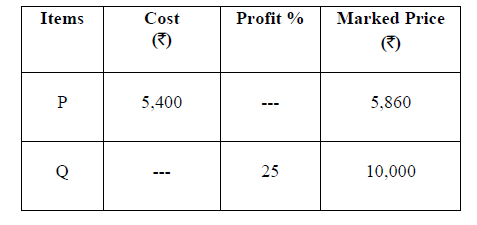# GATE | GATE CS 2021 | Set 1 | Question 7

• Last Updated : 24 May, 2021Details of prices of two items P and Q are presented in the above table. The ratio of cost of item P to cost of item Q is 3:4. Discount is calculated as the difference between the marked price and the selling price. The profit percentage is calculated as the ratio of the difference between selling price and cost, to the cost

`Profit% = ((Selling price – Cost)/Cost)×100 `

The discount on item Q, as a percentage of its marked price, is _______ .
(A) 25
(B) 12.5
(C) 10
(D) 5

Explanation: Given, ratio of cost of item P to cost of item Q is 3:4
So, (3/7)*(total cost of P and Q) = 5400 = cost of P
Total cost of P and Q = 5400*7/3 = 12600
Hence, cost of Q = 12600*4/7 = 7200

Now, the selling price of Q would be
= cost price * (1+profit%) ;as given in table
= 7200 * (1+0.25)
= 7200*1.25
= 9000

(Discount is calculated as the difference between the marked price and the selling price; as given in table.).
Discount %
= (MP – SP) / MP
= (10000 – 9000) / 10000
= 1000/10000
= 1/10
= 0.1 = 10%

Quiz of this Question

My Personal Notes arrow_drop_up
Recommended Articles
Page :